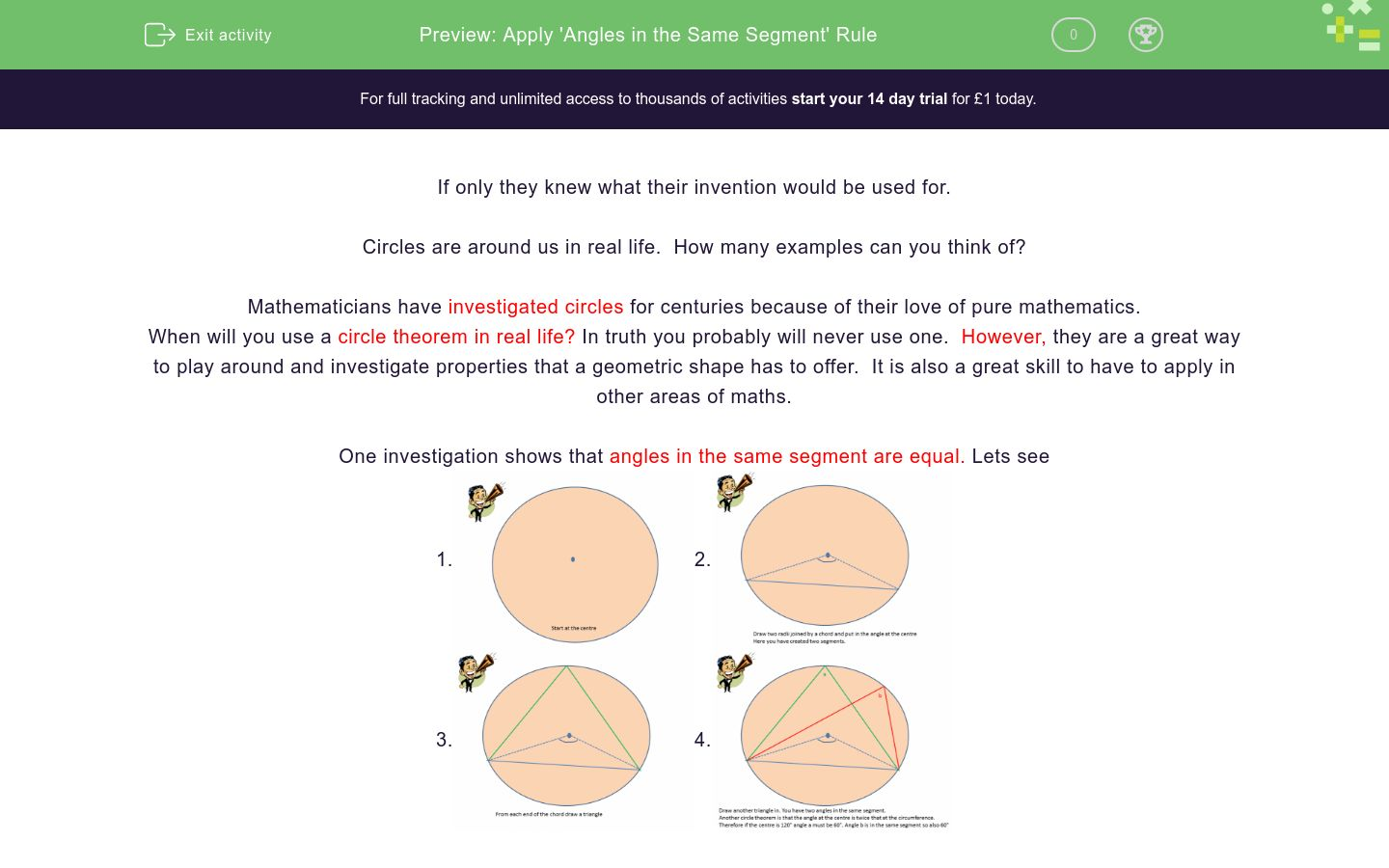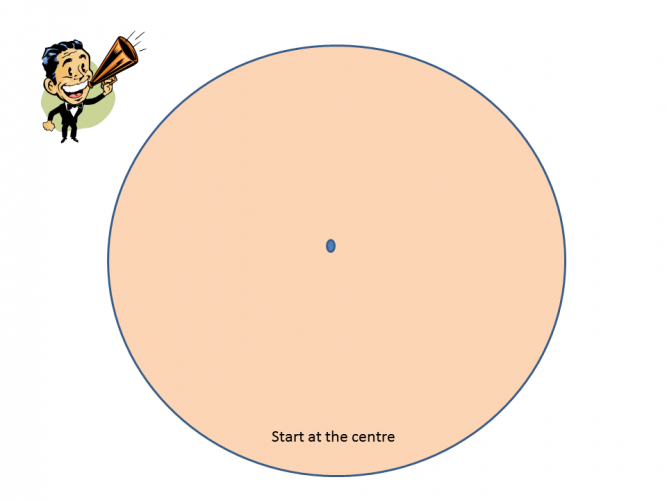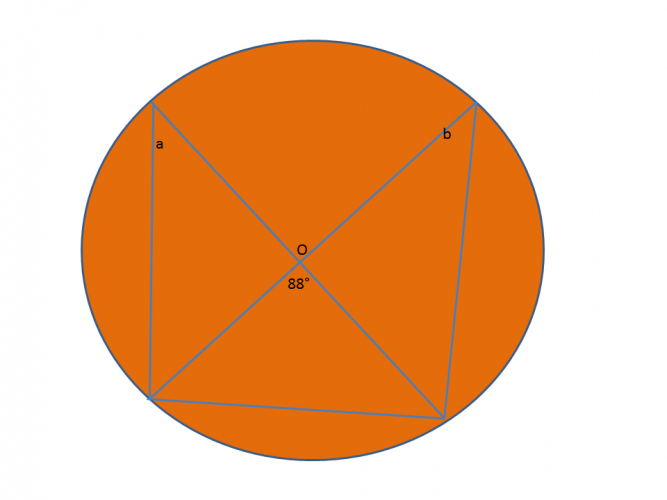# Apply 'Angles in the Same Segment' Rule

In this worksheet, students will learn about angles in the same segment rule.Key stage:  KS 4

GCSE Subjects:   Maths

GCSE Boards:   AQA, Eduqas, Pearson Edexcel, OCR

Curriculum topic:   Geometry and Measures, Basic Geometry

Curriculum subtopic:   Properties and Constructions, Circles

Difficulty level:### QUESTION 1 of 10

If only they knew what their invention would be used for.

Circles are around us in real life.  How many examples can you think of?

Mathematicians have investigated circles for centuries because of their love of pure mathematics.

When will you use a circle theorem in real life? In truth you probably will never use one.  However, they are a great way to play around and investigate properties that a geometric shape has to offer.  It is also a great skill to have to apply in other areas of maths.

One investigation shows that angles in the same segment are equal. Lets see

1.2.3.4.That is all there is to it, or is it?  You are sometimes asked to recall other angle properties.

So roll up, roll up lets go..What is the value of angle a.

47°

43°

133°

180°Find the value of a

Can't tell

66°

48°

114°What is the value of a?64° 18° 116° 46° Angle a is Angle b is40° 86° 94° 47° 68° Angle a is Angle b is Angle c isFind the missing angles.

You will need to recall other angle properties for this one.

 40° 86° 94° 47° 68° Angle a is Angle b is Angle c isFind the value of a.Angle a and b is

88°

44°

92°

46°Find the value of a.

• Question 1What is the value of angle a.

47°
EDDIE SAYS
If you look at the chord and look above it you can see two angles identified by the triangles drawn. We know that angles in the same segment are equal so therefore a = 47°
• Question 2Find the value of a

48°
EDDIE SAYS
These can be a little confusing as there is an angle below 48° Draw in the chord so that you can see that angle a is in the same segment as 48° And as we know the angels are the same.
• Question 3What is the value of a?

36
EDDIE SAYS
Draw the chord in. The angles are in the same segment as they are both above the chord. So therefore the angles are the same. Don't you think that this shapes looks like a bow tie?
• Question 4EDDIE SAYS
If you are every unsure draw in the chord. If you draw it either at the top or the bottom you can see that a is in the same segment as 22° And the added bonus is that there is no working out to do.
• Question 564° 18° 116° 46° Angle a is Angle b is
EDDIE SAYS
Are you getting used to spotting these now? I love this theorem because you are given the answers. Angles in the same segment are always the same.
• Question 640° 86° 94° 47° 68° Angle a is Angle b is Angle c is
EDDIE SAYS
Did I say there was no working out. Okay so occasionally we are going to have to draw on some other knowledge. Angle a + b + 86 = 180° (angles in a triangle) 180 - 86 = 94° ÷ 2 = 47° So angle a and b = 47° Angle c no working out necessary so it is 40°
• Question 7Find the missing angles.

You will need to recall other angle properties for this one.

EDDIE SAYS
Angle a is the tricky one. I will come back to this in a minute. If you draw a chord in you can see that angles b and c are in the same segment so 34 and 78. If you look angle a is not in the same segment as 28°. it is in the same triangle which is why we need to recall other properties. Calculate the missing angle of the triangle with 78° and 34° 180 - 78 - 34 = 68°. You should recall that vertically opposite angles are equal which give you the second angle in the opposite triangle. You now need to recall that angles on a straight line add up to 180° to give us one angle in the triangle with 28° 180 - 68 = 112° Now we are set. 112 + 28 = 140° 180 - 140 = 40°
• Question 8Find the value of a.

42
EDDIE SAYS
Find the missing two angles in the triangle with 94° 180 - 94 = 86° As the triangle is isosceles the the two other angles are 43° Therefore angle a is 43° as it is in the same segment.
• Question 9Angle a and b is

46°
EDDIE SAYS
Here you need to recall the properties that angles on a straight line add up to 180° 180 - 88 = 92° Radii from the centre suggest the triangle is isosceles. 92 ÷ 2 = 46°
• Question 10Find the value of a.

28
EDDIE SAYS
You need to find the angles in the smaller triangle as one of the missing angles is in the same segment as a. Vertically opposite angles are equal. Therefore one missing angle is 92°. Angles in a triangle add up to 180° 180 - 92 - 60 = 28° Therefore angle a is 28°
---- OR ----

Sign up for a £1 trial so you can track and measure your child's progress on this activity.

### What is EdPlace?

We're your National Curriculum aligned online education content provider helping each child succeed in English, maths and science from year 1 to GCSE. With an EdPlace account you’ll be able to track and measure progress, helping each child achieve their best. We build confidence and attainment by personalising each child’s learning at a level that suits them.

Get started